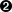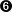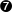#### 6.2.2. The variable definition element

The `variableDef` element is used to define each constant, parameter, or variable used within or generated by the defined subsystem model. It contains attributes including the variable name (used for documentation), an internal and unique `varID` identifier (used for linking inputs, functions and outputs), the units of measure of the variable, and optional axis system, sign convention, alias, and symbol declarations. Optional sub-elements include a written text description and a mathematical description, in MathML-2 content markup, of the calculations needed to derive the variable from other variables or function table outputs. Optional sub-element `isOutput` serves to indicate an intermediate calculation that should be brought out to the rest of the simulation. Another optional sub-element, `isStdAIAA`, indicates the variable name is defined in the AIAA simulation standards document. Another optional sub-element, `uncertainty`, captures the statistical properties of a (normally constant) parameter.

Other optional sub-elements are provided to identify inputs, disturbances, and simulation control parameters, as well as the ability to identify a variable as a state or state derivative for linear model purposes.

There must be a single `variableDef` for each and every input, output or intermediate constant or variable within the DAVEfunc model.

```    variableDef : name, varID, units, [axisSystem, sign, alias, symbol,
initialValue, minValue, maxValue]
description? :
{description character data}
(
provenanceRef : provID
OR
provenance : [provID]
author+ : name, org, [email]
contactInfo* : [contactInfoType, contactLocation]
{text describing contact information}
creationDate : date {in YYYY-MM-DD format}
documentRef* : [docID,] refID
modificationRef* : modID
description?
)?
calculation? :
math {defined in MathML-2 DTD}
(isInput | isControl | isDisturbance)?
isState?
isStateDeriv?
isOutput?
isStdAIAA?
uncertainty? : effect
(normalPDF : numSigmas) | (uniformPDF : bounds+)

```

`variableDef` attributes:

A UNICODE name for the variable (may be the same string as the `varID`).

`varID`

An internal identifier that is unique within the file.

`units`

An optional indicator of the axis system (body, inertial, etc.) in which the signal is measured. See [AIAA11] or Section 6.5, “Conventions” below for recommended practice for nomenclature.

An optional indicator of which direction is considered positive (+RWD, +UP, etc.). See [AIAA11] or the section on Section 6.5, “Conventions” below for recommended practice for abbreviations.

An optional, facility-specific variable name, perhaps used in the equations of motion or control system model, that does not conform to the AIAA standard for variable names. Use of this attribute is discouraged for portability reasons.

`symbol`

A UNICODE Greek symbol for the signal [to be superseded with more formal MathML or TeX element in a later release].

`initialValue`

An optional initial value for the parameter. This is normally specified for constant parameters only.

`minValue`

An optional minimum value that the variable may take on, regardless of how that value is determined. If present, this attribute implies a one-sided minimum value limiter that is applied to the final value of the variable. If the minimum value is greater than a sibling `maxValue` attribute the result is undetermined. This attribute must be a string representing a constant numeric value.

`maxValue`

An optional maximum value that the variable may take on, regardless of how that value is determined. If present, this attribute implies a one-sided maximum value limiter that is applied to the final value of the variable. If the maximum value is lesser than a sibling `minValue` attribute the result is undetermined. This attribute must be a string representing a constant numeric value.

`variableDef` sub-elements:

An optional text description of the variable.

The optional provenance element allows the author to describe the source and history of the data within this `variableDef`. Alternatively, a `provenanceRef` reference can be made to a previously defined `provenance`.

An optional container for the MathML-2 content markup that describes how this variable is calculated from other variables or function table outputs. This element contains a single `math` element which is defined in the MathML-2 markup language.

A MathML-2 calculation can include both constants (using the content numeric `cn` element) and references to other variables internal to the parent `DAVEfunc` description. The variables (which can include the output, or dependent variable of a `function` table) are identified using its `varID` attribute string in the appropriate MathML content identifier (`ci`) element of the expression.

Examples of MathML expressions appear later in this document.

This optional element, if present, signifies that this variable is an input to the model, such as a pilot inceptor deflection or Mach number. Useful for linear model extraction tools. It must not be the result of a calculation or be cited as the dependent variable of a function.

This optional element, if present, signifies that this variable is a simulation control parameter, such as a trim flag or simulation time step measurement. Simulation control parameters should have no influence on the dynamic behavior of the model and should be ignored by a linear model extraction tool.

This optional element, if present, signifies that this variable represents an external disturbance input to the model; this is useful for linear model extraction tools to partition this input separately from the other model inputs.

This optional element, if present, signifies that this variable needs to be passed as an output. How this is accomplished is up to the implementer. Unless specified by this element, a variable is considered an output only if it is the result of a calculation or function AND is not used elsewhere in the `DAVEfunc`.

This optional element, if present, signifies that this variable is one of the standard AIAA simulation variable names that are defined as Annex A to [AIAA11]. Such identification should make it easier for the importing process to connect this variable (probably an input or output of the model) to the appropriate variable to/from the user's simulation framework.

This optional element, if present, signifies that this variable serves as a state of the model.

This optional element, if present, signifies that this variable serves as a state derivative of the model.

This optional element, if present, describes the uncertainty of this parameter. See the section on Statistics below for more information about this element.

Example 2. An example of two `variableDef` elements defining input signals

```<!--                     ==========================                     -->
<!-- ====================   VARIABLE DEFINITIONS   ==================== -->
<!--                     ==========================                     -->

<!-- ================== -->
<!--  Input variables   -->
<!-- ================== -->

<variableDef name="mach"varID="XMACH"units="nd" symbol="M">
<description>
Mach number (dimensionless)
</description>
<isInput/><isStdAIAA/></variableDef>
.
.
.
<variableDef name="dbfll" varID="DBFLL" units="deg"sign="TED"symbol="&#x3B4;bfll">
<description>
Lower left body flap deflection, deg, positive trailing-edge-down (so deflections are
always zero or positive).
</description>
<isInput/>
</variableDef>
```The `name` attribute is intended for humans to read, perhaps as the signal name in a wiring diagram. Note that "machNumber" is one of the standard AIAA simulation variable names.The `varID` attribute is intended for the processing application to read. This is an internal identifier that must be unique within this model.The optional `isInput` attribute indicates this variable should be treated as an input to the model for model hierarchy and linear model extraction (for example).The optional `isStdAIAA` sub-element indicates this signal is one of the predefined standard variables that most simulation facilities define in their equations of motion code. The `name` attribute should correspond to the standard AIAA parameter name from Annex A of [AIAA11] or subsequent standards documentThe mandatory `units` attribute describes the units of measure of the variable. See Section 6.5.6, “Units” below for a recommended list of units-of-measure abbreviations.The optional `sign` attribute describes the sign convention that applies to this variable. In this case, the lower-left body-flap is positive with trailing-edge-down deflection. See Section 6.5.5, “Sign conventions” below for a recommended list of sign abbreviations.The optional `symbol` attribute allows a UNICODE character string that might be used for this variable in a symbols listing.

Example 3. A simple local variable definition example

This DAVE-ML excerpt defines `CLBFLLO` which is the dependent variable (output) from a table lookup function, shown later in Example 11. It is subsequently used in the calculation of the lower-left body flap lift coefficient, shown in Example 4.

```      <!-- ================== -->
<!--  Local variables   -->
<!-- ================== -->

<!-- PRELIMINARY BUILDUP EQUATIONS -->

<!--  LOWER LEFT BODY FLAP CONTRIBUTIONS -->

<!--    table output signal   -->
<variableDef name="CLdbfll_0" varID="CLBFLL0" units="nd">
<description>
Output of CLBFLL0 function; lift force contribution of
lower left body flap deflection due to alpha^0 (constant
term).
</description>
</variableDef>
```

Since this signal is not flagged as an input, control, disturbance or output, this variable is an intermediate signal local to this model.

Example 4.  A more complete `variableDef` example with a `calculation` element

In this example, the local variable `CLBFLL` is defined as a calculated quantity, based on several other input or local variables including the CLBFLL0 function output variable defined in the previous example. Note the `description` element is used to describe the equation in Fortran-ish human-readable text. The `calculation` element describes this same equation in MathML-2 content markup syntax; this portion should be used by parsing applications to create either source code, documentation, or run-time calculation structures.

```<!--    lower left body flap lift buildup -->
<variableDef name="CLdbfll" varID="CLBFLL" units="nd">
<description>
Lift contribution of lower left body flap deflection
CLdbfll = CLdbfll_0 + alpha*(CLdbfll_1 + alpha*(CLdbfll_2
+ alpha*CLdbfll_3))</description>
<calculation><math xmlns="http://www.w3.org/1998/Math/MathML">
<apply><plus/>
<ci>CLBFLL0</ci><apply>
<times/>
<ci>ALP</ci>
<apply>
<plus/>
<ci>CLBFLL1</ci>
<apply>
<times/>
<ci>ALP</ci>
<apply>
<plus/>
<ci>CLBFLL2</ci>
<apply><times/>
<ci>ALP</ci>
<ci>CLBFLL3</ci>
</apply> <!--            a*c3   --></apply> <!--        (c2 + a*c3)  -->
</apply> <!--        a*(c2 + a*c3)  -->
</apply> <!--    (c1 + a*(c2 + a*c3)) -->
</apply> <!--    a*(c1 + a*(c2 + a*c3)) -->
</apply> <!-- c0 + a*(c1 + a*(c2 + a*c3)) -->
[/itex]
</calculation>
</variableDef>
```This Fortran-ish equation, located in the `description` element, is provided in this example for the benefit of human readers; it should not parsed by the processing application.A `calculation` element always embeds a MathML-2 `math` element; note the definition of the MathML-2 namespace.Each `apply` tag pair surrounds a math operation (in this example, a `plus` operator) and the arguments to that operation (in this case, a variable `CLBFLL` defined elsewhere is added to the results of the nested `apply` operation).The content identifier (`ci`) MathML-2 element gives the `varID` of the previously defined variables used in this equation; this variable represents the output of the `CLBFLL0` function found in Example 11 that is captured in the `CLBFLL0` variable defined in Example 3. The other `ci` elements are not defined in this manual but are defined in the full model.Inner-most `apply` multiplies variables `ALP` and `CLBFLL3`.The comments here are useful for humans to understand how the equation is being built up; the processing application ignores all comments.

Example 5. Another example of an output variable based on a `calculation` element

```
<!-- ================== -->
<!--  Output variables  -->
<!-- ================== -->

<variableDef name="CL" varID="CL" units="nd" sign="+UP" symbol="CL">
<description>
Coefficient of lift
CL = CL0 + CLBFUL + CLBFUR + CLBFLL + CLBFLR +
CLWFL + CLWFR + CLRUD + CLGE + CLLG
</description>
<calculation>
<math xmlns="http://www.w3.org/1998/Math/MathML">
<apply><plus/>
<ci>CL0</ci>
<ci>CLBFUL</ci>
<ci>CLBFUR</ci>
<ci>CLBFLL</ci><ci>CLBFLR</ci>
<ci>CLWFL</ci>
<ci>CLWFR</ci>
<ci>CLRUD</ci>
<ci>CLGE</ci>
<ci>CLLG</ci>
</apply>
[/itex]
</calculation>
<isOutput/></variableDef>
```Here `apply` simply sums the value of these variables, referenced by their `varID`s.This `ci` element refers to the lower left body flag lift contribution calculated in the previous example.The `isOutput` element signifies to the processing application that this variable should be made visible to models external to this `DAVEfunc`.

Example 6. An intermediate variable with a `calculation` element that uses a DAVE-ML function extension to the default MathML-2 function set

```
<!-- ================== -->
<!--    ATAN2 example   --><!-- ================== -->

<variableDef name="Wind vector roll angle" varID="PHI" units="rad">
<description>
This encodes the equation PHI = atan2( tan(BETA), sin(ALPHA) ) where atan2
is the two-argument arc tangent function from the ANSI C standard math
library.
</description>
<calculation>
<math xmlns="http://www.w3.org/1998/Math/MathML">
<apply>
<csymbol definitionURL="http://daveml.org/function_spaces.html#atan2"
encoding="text">atan2
</csymbol>
<apply>
<tan/>
<ci>BETA</ci></apply>
<apply>
<sin/>
<ci>ALPHA</ci></apply>
</apply>
[/itex]
</calculation>

</variableDef>
```This excerpt shows how to calculate wind roll angle, phi, from angle-of-attack and angle-of-sideslip; it comes from the Apollo aerodynamics data book [NAA64].The `csymbol` element is provided by MathML-2 as a means to extend the function set of MathML-2. An extension for atan2 is the only function defined at present but others may be added to the set in the future. Note the specific URI that uniquely identifies this function; it is also the URL (web address) of the documentation of the interpretation of the atan2 function.BETA is the `varID` of a previously defined variable.ALPHA is the `varID` of a previously defined variable.

2011-03-31